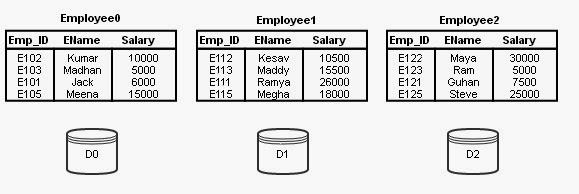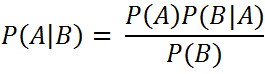`  I request GUEST WRITERS for this web site. Anyone interested please contact me at saravu2k2013@gmail.com Thanks  `

## Range Partitioning Sort

#### Assumptions:

Assume n processors, P0, P1, …, Pn-1 and n disks D0, D1, …, Dn-1.
Disk Di is associated with Processor Pi.
Relation R is partitioned into R0, R1, …, Rn-1 using Round-robin technique or Hash Partitioning technique or Range Partitioning technique (if range partitioned on some other attribute other than sorting attribute)

#### Objective:

Our objective is to sort a relation (table) Ri that resides on n disks on an attribute A in parallel.

#### Steps:

Step 1: Partition the relations Ri on the sorting attribute A at every processor using a range vector v. Send the partitioned records which fall in the ith range to Processor Pi where they are temporarily stored in Di.
Step 2: Sort each partition locally at each processor Pi. And, send the sorted results for merging with all the other sorted results which is trivial process.

#### Point to note:

Range partition must be done using a good range-partitioning vector. Otherwise, skew might be the problem.

#### Example:

Let us explain the above said process with simple example.Consider the following relation schema Employee;

Employee (Emp_ID, EName, Salary)
Assume that relation Employee is permanently partitioned using Round-robin technique into 3 disks D0, D1, and D2 which are associated with processors P0, P1, and P2. At processors P0, P1, and P2, the relations are named Employee0, Employee1 and Employee2 respectively. This initial state is given in Figure 1.Figure 1

Assume that the following sorting query is initiated.
SELECT * FROM Employee ORDER BY Salary;
As already said, the table Employee is not partitioned on the sorting attribute Salary. Then, the Range-Partitioning technique works as follows;

Step 1:
At first we have to identify a range vector v on the Salary attribute. The range vector is of the form v[v0, v1, …, vn-2]. For our example, let us assume the following range vector;
v[14000, 24000]
This range vector represents 3 ranges, range 0 (14000 and less), range 1 (14001 to 24000) and range 2 (24001 and more).
Redistribute the relations Employee0, Employee1 and Employee2 using these range vectors into 3 disks temporarily. After this distribution disk 0 will have range 0 records (i.e, records with salary value less than or equal to 14000), disk 1 will have range 1 records (i.e, records with salary value greater than 14000 and less than or equal to 24000), and disk 2 will have range 2 records (i.e, records with salary value greater than 24000).
This redistribution according to range vector v is represented in Figure 2 as links to all the disks from all the relations. Temp_Employee0, Temp_Employee1, and Temp_Employee2, are the relations after successful redistribution. These tables are stored temporarily in disks D0, D1, and D2. (They can also be stored in Main memories (M0, M1, M2) if they fit into RAM).Figure 2

Step 2:
Now, we got temporary relations at all the disks after redistribution.
At this point, all the processors sort the data assigned to them in ascending order of Salary individually. The process of performing the same operation in parallel on different sets of data is called Data Parallelism.
Final Result:
After the processors completed the sorting, we can simply collect the data from different processors and merge them. This merge process is straightforward as we have data already sorted for every range. Hence, collecting sorted records from partition 0, partition 1 and partition 2 and merging them will give us final sorted output.